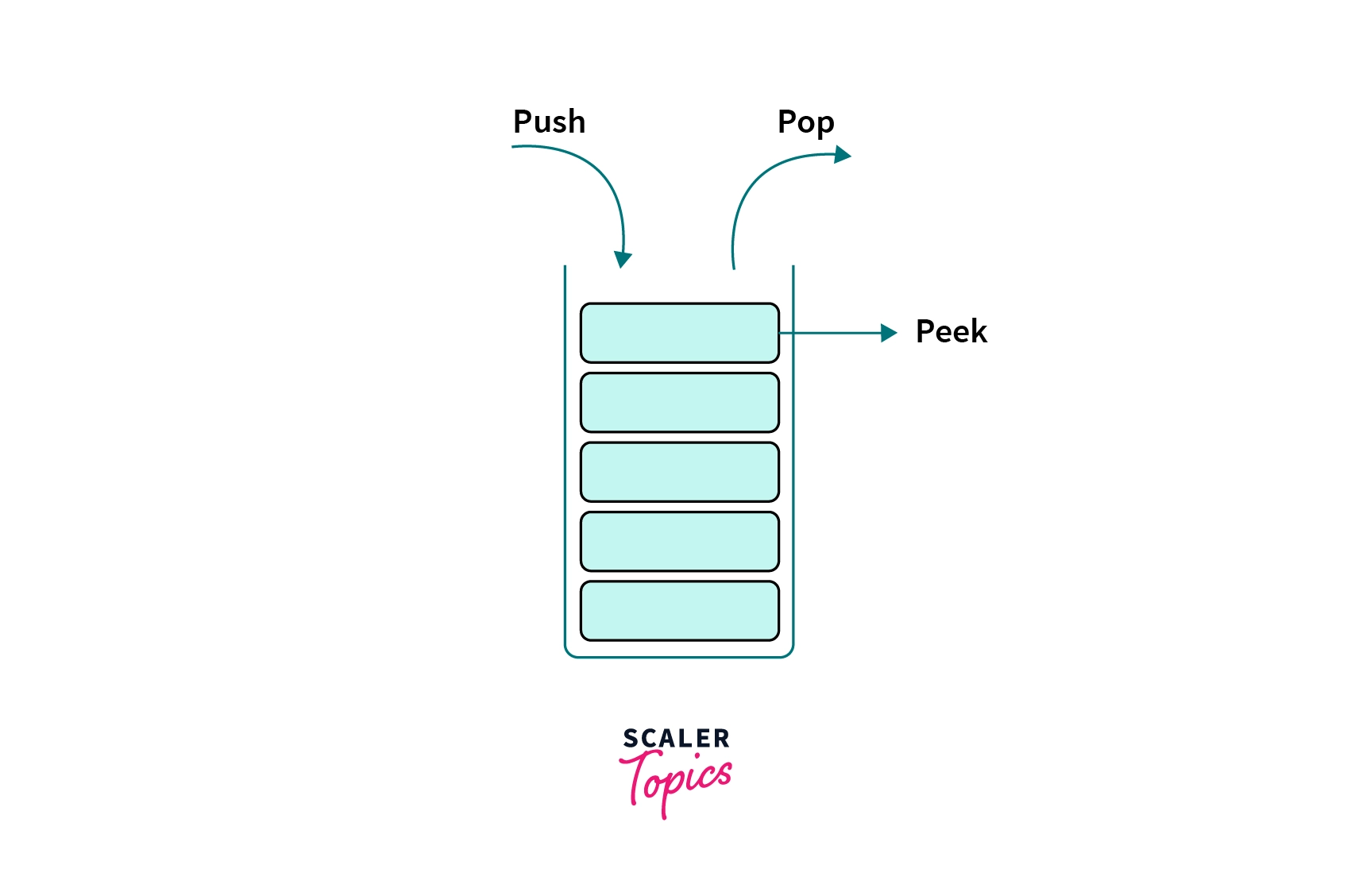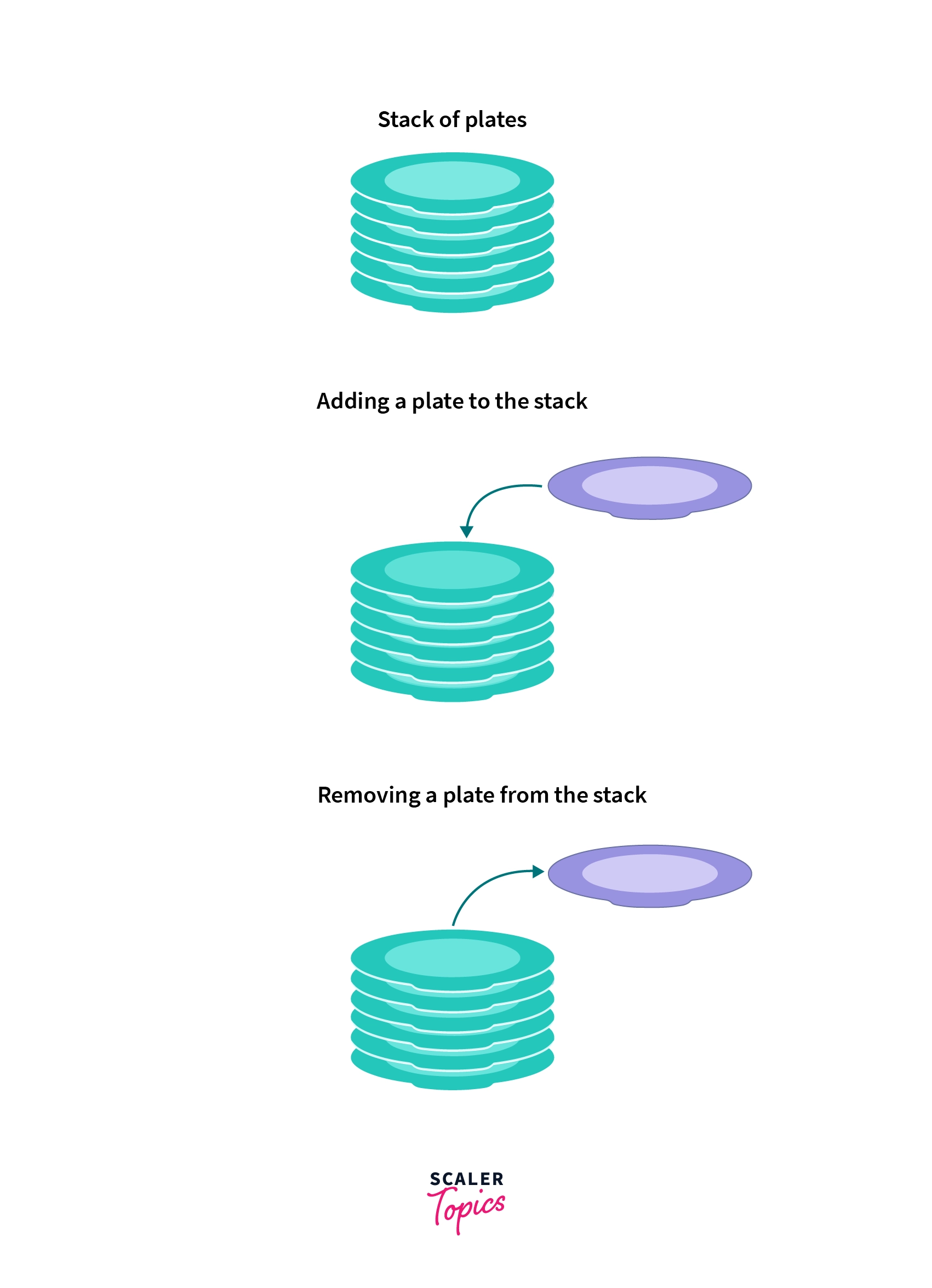# Stack in CChallenge Inside! : Find out where you stand! Try quiz, solve problems & win rewards!

## Learn via video courseFree C++ Course: Learn the Essentials
By Prateek NarangFree
5Enrolled: 26844Free C++ Course: Learn the Essentials
Prateek NarangFree
5
Enrolled: 26844

## Overview

In C, a Stack is a linear data structure that follows the LIFO (Last In First Out) approach to perform a series of basic operations like push, pop, peek, and traverse. A Stack can be implemented using an Array or Linked List.

## Scope of Article

• All the operations of the Stack Data Structure will be explained thoroughly while discussing their time complexities in this article.
• We will learn how to implement a static stack using arrays and a dynamic stack using linked lists in this article.

## Introduction to Stack in C

A Stack is an abstract linear data structure serving as a collection of elements that are inserted (push operation) and removed (pop operation) according to the Last in First Out (LIFO) approach. Insertion and deletion happen on the same end (top) in a Stack. The top of the stack is returned using the peek operation.Notes: An abstract data type has some associated operations (like Push and Pop in stack), while its representation remains hidden.

## What is the Stack Data Structure in C?

In C, the Stack data structure is an ordered, linear sequence of items. It is a LIFO (Last In First Out) data structure, which means that we can insert or remove an item at the top of the stack only. It is a sequential data type, unlike an array. In an array, we can access any of its elements using indexing, but we can only access the top most element in a stack. The name "Stack" for this data structure comes from the analogy to a set of physical items stacked on top of each other. An example of this data structure would be a stack of plates: We can only add a plate to the top of the stack and take a plate from the top of the stack. A stack of coins or a stack of books can also be a real-life example of the stack data structure.There are two types of Stack data structure: Static and Dynamic

### Static Stack

A Static Stack (also known as a bounded stack) has a bounded capacity. It can contain a limited number of elements. If a static stack is full and does not have any space remaining for another element to be pushed to it, it is then called to be in an Overflow State.

In C, a static stack is implemented using an Array, as arrays are static.

### Dynamic State

A Dynamic Stack is a stack data structure whose capacity (maximum elements that can be stored) increases or decreases in runtime, based on the operations (push or pop) performed on it.

In C, a dynamic stack is implemented using a Linked List, as linked lists are dynamic data structures.

## How does Stack Work in C?

In C, the stack data structure works using the LIFO (Last In First Out) approach. Initially, we set a Peek pointer to keep track of the topmost element of the stack. Then the stack is initialized to -1 as its peek, as we add (push) elements to the stack, the peek gets updated to point to its topmost element, and if we remove (pop) elements from the stack, the peek gets reduced.
We use stack to perform its main two operations: Push and Pop, other operations being Peek, isEmpty and isFull.

### Push Operation

We use the Push operation to add an element to the top of the stack.

### Pop Operation

We use the Pop operation to return and remove the topmost element of the stack.

### Peek Operation

We use the Peek Operation to display the topmost element of the stack.

### IsEmpty Operation

We use the IsEmpty Operation to check whether the stack is empty or not.

### IsFull Operation

We can use the IsFull Operation to check whether the stack is full or not.

This operation can only be used with the static implementation of the stack (using an array), also called a bounded stack.

## Time Complexity of Stack Operations

The time complexity of the several stack operations are:

### Push Operation: O(1)

The time complexity of the Pop operation would be O(1) as in this operation, we are inserting an element at the top of the stack only.

### Pop Operation: O(1)

The time complexity of the Push operation would be O(1) as in this operation, we are removing and returning an element from the top of the stack only.

### Peek Operation: O(1)

The time complexity of the Peek operation would be O(1) as in this operation, we are returning only the topmost element of the stack.

### IsEmpty Operation: O(1)

The time complexity of the IsEmpty operation would be O(1) as in this operation, we are checking whether the topmost element is null or not.

### IsFull Operation: O(1)

The time complexity of the IsFull operation would be O(1) as in this operation, we are checking whether the topmost element is at the maximum position or not.

## Implementing Stack in C

In C, we can implement the Stack data structure using an array or a linked list.

### Stack Program in C using Array

We will be using an array to implement a Static Stack (Bounded Stack) as arrays are static in C.
A Static Stack has a bounded capacity. A static stack is called to be in an Overflow State if it is full (no elements can be further pushed into it).

Implementation

Output

In the above example, we are implementing a static stack with a capacity of 1000 in C using an array. The static stack performs the following operations: Push, Pop, Peek, isEmpty, and isFull. The program stops when the user chooses 0 as an option.

### Stack Program in C using Linked List

We will be using a linked list to implement a Dynamic Stack as linked lists are dynamic data stuctures.
A Dynamic Stack does not have a bounded capacity, we can push as much as elements as we want into it.

Implementation:

Output

In the above example, we are implementing a dynamic stack in C using a linked list. The dynamic stack performs the following operations: Push, Pop, Peek, and isEmpty. The program stops when the user chooses $0$ as an option.

## Applications of Stack in C

• Expression Conversion
1. Infix to Postfix
2. Infix to Prefix
3. Postfix to Infix
4. Prefix to Infix
• Recursion: Call Stack
Recursive functions use something called the "call stack". When a program calls a function, the function is set at the top of the call stack. The last function call solves first as it is at the top of the stack, and the first function call is solved at last.
• Memory Management: Stack allocation
The stack allocation happens on continuous blocks of the memory. It is known as stack memory allocation because the allocation is happening in the function call stack. The size of memory to be allocated is known to the compiler and whenever a function is called, its variables get memory allocated on the stack. The memory for the variables is de-allocated whenever the function call is over.
• Compilation: Syntax Parsing
Many compilers use a stack data structure for parsing the syntax for expressions, blocks of code, etc., before translating the high-level code into low-level code.
• Expression Evaluation: Parenthesis Checking
The stack data structure is used in expression evaluation as it keeps the record of the opening and closing parenthesis.

## Conclusion

• A Stack is a linear abstract data structure.
• The stack data structure can be of two types: static and dynamic stack.
• A static stack is implemented using an array in C, whereas a dynamic stack is implemented using a linked list in C.
• Push and Pop are the two primary operations of a stack, others being Peek, isEmpty and isFull.
• Stack can be used in expression conversion, like, infix to postfix, infix to prefix, postfix to infix, and prefix to infix.
• The time complexity of all the operations of a Stack is O(1).